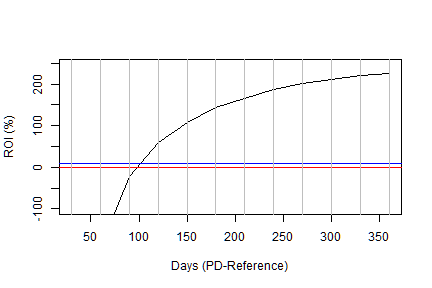Payday lending – how low can they go?

A post from TMM referenced the British payday lender wonga. As this is a way of credit which is either uncommon or illegal in Germany, a word about their business model: They give out very small credits (upper limit 400 pound) for a short time, up to 30 days. For this they will charge 5.5 pounds in fees and roughly 1% interest per day, based on a yearly rate of 365% .

Nice business, if you can get it. What peeked my interest was the question: How much losses can they take on these loans and still make a decent return on investment? After fiddling around with the numbers I pulled from thin air, the result is: They can troll the bottom of the sea, and still make a living. Here is my reasoning:

I simulate a credit portfolio of 100,000 credits within a business year of 365 days, ignoring weekends and holidays. Loan size and duration are uniformly distributed within the limits set by wonga. I assign a probability of default of between 80% and 100%. I will define the time reference, in which this probability applies, in the next step. So this value can be interpreted as “the creditor will go bust within the next (x) days with (y) likelihood”. I further assume an equity of 1 mil pounds to start the business. This ensures that the lender himself does not get in the red.

numYears =1
numCost = 100000*numYears
numDays = 365*numYears
equity = 1e6

credits = data.frame(id = 1:numCost,
loan = round(runif(numCost, min=1, max =400),0),
duration = as.integer(runif(numCost, min=1, max = 30)),
loanday = as.integer(runif(numCost,min=1,max=numDays)),
pd = runif(numCost, min = 0.8, max = 1))

credits$repayday = credits$loanday + credits$duration  I calculate the value of the outstanding amount on the repayment day as the size of the loan + 1% interest per day of duration. I assume that the fixed amount of 5.50 pounds coveres the fixed costs of the credit. To calculate the actual repayment made to the lender I make a random draw to simulate the possibility of default, where the base probability of default is wheighted with the duration of the credit in reference the time reference chosen for the probability of default. Usually this is one year, but I will vary it in the analysis. In the next step I calculate the cashflow and the balance sheet during the business year, and calculate the return on investment as the value of the balance sheet at the end of the year, divided by the equity. calcRoi = function(credits, defaultDenum=365) { credits$repayment = (credits$loan* (1+credits$duration/100))*
rbinom(numCost, 1, (1-credits$pd*credits$duration/defaultDenum))

cashflow = data.frame(day = 1:numDays, outflow = 0, inflow = 0, balance = 0)
cashflow$balance= equity for(day in 1:numDays) { cashflow$outflow[day] = sum(credits$loan[which(credits$loanday == day)])
cashflow$inflow[day] = sum(credits$repayment[which(credits$repayday == day)]) if(day>1) { cashflow$balance[day]=cashflow$balance[day-1]+cashflow$inflow[day]-cashflow$outflow[day] } } (ROI = cashflow$balance[nrow(cashflow)]/equity)
}


I now calculate the ROI for different time references in the range from 1 month to 1 year and plot the resultThe red line is the break-even and the blue line marks 10% ROI.

As you can see, the payday lender will make a decent ROI of 10% even with an average probability of default of 90% within the next 3-4 months, even if he writes off the defaulted loans completely. The crucial part of the business thus will be walking the fine line of selecting creditors who are nearly busted, so they are desperate enough to apply to this loan, but not yet busted, so that the lender will lose on a too large part of the credit portfolio.